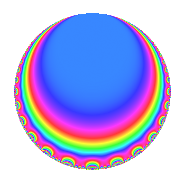Properties

 Label 3600.1.l.bLevel 3600 Weight 1 Character orbit 3600.l Analytic conductor 1.797 Analytic rank 0 Dimension 2 Projective image $$S_{4}$$ CM/RM No Inner twists 2

Related objects

Newspace parameters

 Level: $$N$$ = $$3600 = 2^{4} \cdot 3^{2} \cdot 5^{2}$$ Weight: $$k$$ = $$1$$ Character orbit: $$[\chi]$$ = 3600.l (of order $$2$$ and degree $$1$$)

Newform invariants

 Self dual: No Analytic conductor: $$1.79663404548$$ Analytic rank: $$0$$ Dimension: $$2$$ Coefficient field: $$\Q(\sqrt{-2})$$ Coefficient ring: $$\Z[a_1, \ldots, a_{11}]$$ Coefficient ring index: $$1$$ Projective image $$S_{4}$$ Projective field Galois closure of 4.2.10800.2 Artin image size $$48$$ Artin image $\GL(2,3)$ Artin field Galois closure of 8.2.5598720000.2

$q$-expansion

Coefficients of the $$q$$-expansion are expressed in terms of $$\beta = \sqrt{-2}$$. We also show the integral $$q$$-expansion of the trace form.

 $$f(q)$$ $$=$$ $$q$$ $$+ q^{7}$$ $$+O(q^{10})$$ $$q$$ $$+ q^{7}$$ $$-\beta q^{11}$$ $$+ q^{13}$$ $$-\beta q^{17}$$ $$- q^{19}$$ $$-\beta q^{23}$$ $$+ \beta q^{29}$$ $$- q^{31}$$ $$- q^{43}$$ $$-\beta q^{47}$$ $$+ \beta q^{59}$$ $$+ q^{61}$$ $$+ q^{67}$$ $$-\beta q^{77}$$ $$+ \beta q^{83}$$ $$+ q^{91}$$ $$+ q^{97}$$ $$+O(q^{100})$$ $$\operatorname{Tr}(f)(q)$$ $$=$$ $$2q$$ $$\mathstrut +\mathstrut 2q^{7}$$ $$\mathstrut +\mathstrut O(q^{10})$$ $$2q$$ $$\mathstrut +\mathstrut 2q^{7}$$ $$\mathstrut +\mathstrut 2q^{13}$$ $$\mathstrut -\mathstrut 2q^{19}$$ $$\mathstrut -\mathstrut 2q^{31}$$ $$\mathstrut -\mathstrut 2q^{43}$$ $$\mathstrut +\mathstrut 2q^{61}$$ $$\mathstrut +\mathstrut 2q^{67}$$ $$\mathstrut +\mathstrut 2q^{91}$$ $$\mathstrut +\mathstrut 2q^{97}$$ $$\mathstrut +\mathstrut O(q^{100})$$

Character Values

We give the values of $$\chi$$ on generators for $$\left(\mathbb{Z}/3600\mathbb{Z}\right)^\times$$.

 $$n$$ $$577$$ $$901$$ $$2801$$ $$3151$$ $$\chi(n)$$ $$1$$ $$1$$ $$-1$$ $$1$$

Embeddings

For each embedding $$\iota_m$$ of the coefficient field, the values $$\iota_m(a_n)$$ are shown below.

For more information on an embedded modular form you can click on its label.

Label $$\iota_m(\nu)$$ $$a_{2}$$ $$a_{3}$$ $$a_{4}$$ $$a_{5}$$ $$a_{6}$$ $$a_{7}$$ $$a_{8}$$ $$a_{9}$$ $$a_{10}$$
1601.1
 1.41421i − 1.41421i
0 0 0 0 0 1.00000 0 0 0
1601.2 0 0 0 0 0 1.00000 0 0 0
 $$n$$: e.g. 2-40 or 990-1000 Significant digits: Format: Complex embeddings Normalized embeddings Satake parameters Satake angles

Inner twists

Char. orbit Parity Mult. Self Twist Proved
1.a Even 1 trivial yes
3.b Odd 1 yes

Hecke kernels

This newform can be constructed as the kernel of the linear operator $$T_{7}$$ $$\mathstrut -\mathstrut 1$$ acting on $$S_{1}^{\mathrm{new}}(3600, [\chi])$$.